Search

Graphing Quadratic Functions: Examples (page 4 of 4)

Sections: Introduction, The meaning of the leading coefficient / The vertex, Examples• Find the x-intercepts and vertex of y = x2  4x + 2.

Since it is so simple to find the y-intercept (and it will probably be a point in my T-chart anyway), they are only asking for the x-intercepts this time. To find the x-intercept, I set y equal 0 and solve:

0 = x2  4x + 2

x2 + 4x  2 = 0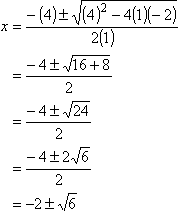For graphing purposes, the intercepts are at about (4.4, 0) and (0.4, 0). (When I write down the answer, I will of course use the "exact" form, with the square roots; my calculator's decimal approximations are just for helping me graph.)

To find the vertex, I look at the coefficients: a = 1 and b = 4. Then:

h = (4)/2(1) = 2

To find k, I plug h = 2 in for x in y = x2  4x + 2, and simplify:

k = (2)2  4(2) + 2 = 4 + 8 + 2 = 10  4 = 6

Now I'll find some additional plot points, to help me fill in my graph: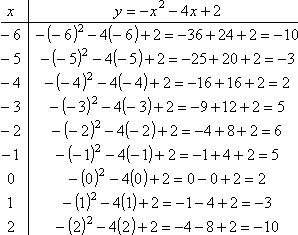Note that I picked x-values that were centered around the x-coordinate of the vertex. Now I'll plot the parabola: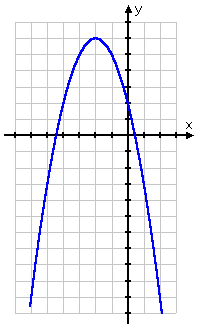The vertex is at (2, 6), and the intercepts are at the following points:

(0, 2)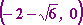, and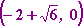• Find the x-intercepts and vertex of y = x2 + 2x  4.

To find the vertex, I look at the coefficients: a = 1 and b = 2. Then:

h = (2)/2(1) = 1

To find k, I'll plug h in for x and simplify:

k = (1)2 + 2(1)  4 = 1 + 2  4 = 2  5 = 3

The vertex is below the x-axis, and, since this is a negative quadratic, I know that the parabola is going to be upside-down. So can my line possibly cross the x-axis? Can there possibly be any x-intercepts? Of course not! So I expect to get "no (real) solution" when I try to find the x-intercepts, but I need to show my work anyway. To find the x-intercept, I set y equal 0 and solve:

0 = x2 + 2x  4

x2  2x + 4 = 0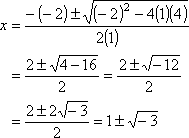As soon as I get a negative inside the square root, I know that I can't get a graphable solution. So, as expected, there are no x-intercepts. Now I'll find some additional plot points, to fill in my graph: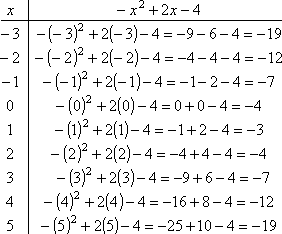Note that I picked x-values that were centered around the x-coordinate of the vertex. Now I'll plot the parabola:   Copyright © Elizabeth Stapel 2002-2011 All Rights Reserved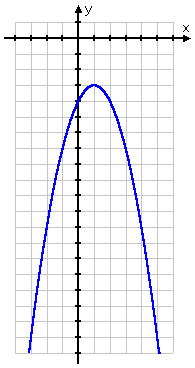The vertex is at (1, 3), and the only intercept is at (0, 4).

This last exercise illustrates one way you can cut down a bit on your work. If you solve for the vertex first, then you can easily tell if you need to continue on and look for the x-intercepts, or if you can go straight on to plotting some points and drawing the graph. If the vertex is below the x-axis (that is, if the  y-value is negative) and the quadratic is negative (so the parabola opens downward), then there will be no x-intercepts. Similarly, if the vertex is above the x-axis (that is, if the y-value is positive) and the quadratic is positive (so the parabola opens upward), then there will be no x-intercepts.

In most of the graphs that I did (though not the first one), it just so happened that the points on the T-chart were symmetric about the vertex; that is, that the points "matched" on either side of the vertex. While a parabola is always symmetric about the vertical line through the vertex (the parabola's "axis"), the T-chart points might not be symmetric. In particular, the T-chart points will not "match" if the x-coordinate of the vertex is something other than a whole number or a half-number (such as "3.5"). Warning: Don't expect the plot-points always to "match up" on either side of the vertex; in particular, don't do half the points on your T-chart and then "fill in" the rest of your T-chart by assuming a symmetry that might not exist.

Other tips for graphing: If the parabola is going to be "skinny", then expect that you will get some very large values in your T-chart. You will either end up with a really tall graph or else a rather short T-chart. If the parabola is going to be "fat", then expect that you will probably have to plot points with fractions as coordinates. In either case, when you go to connect the dots to draw the parabola, you might find it helpful to turn the paper sideways and first draw the really curvy part through the vertex, making sure that it looks nice and round. Then turn the paper back right-side-up and draw the "sides" of the parabola.

Warning: Draw your graphs big enough to be clearly seen by your instructor. If you're fitting more than two or maybe three graphs on one side of a standard sheet of paper, then you're drawing your graphs way too small.

<< Previous  Top  |  1 | 2 | 3 | 4  |  Return to Index

 Cite this article as: Stapel, Elizabeth. "Graphing Quadratic Functions: Examples." Purplemath. Available from     https://www.purplemath.com/modules/grphquad4.htm. Accessed [Date] [Month] 2016

Reviews of
Internet Sites:
Free Help
Practice
Et Cetera

Study Skills Survey

Tutoring from Purplemath
Find a local math tutor